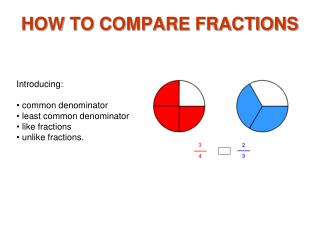DownloadDownload PresentationHOW TO COMPARE FRACTIONS

# HOW TO COMPARE FRACTIONS

Download Presentation## HOW TO COMPARE FRACTIONS

- - - - - - - - - - - - - - - - - - - - - - - - - - - E N D - - - - - - - - - - - - - - - - - - - - - - - - - - -
##### Presentation Transcript

1. HOW TO COMPARE FRACTIONS • Introducing: • common denominator • least common denominator • like fractions • unlike fractions.

2. Compare Fractions 1 The fractions 3/4 and 2/4 have the same denominator. Fractions with the same denominators are like fractions.

3. Compare Fractions 2 If denominators are the same, the fraction with the larger numerator is larger. So 3/4 is larger than 2/4 .

4. Compare Fractions 3 9/16 and 7/16 are like fractions. The numerator 9 in 9/16 is larger than the numerator 7 in 7/16, making 9/16 larger than 7/16 .

5. Compare Fractions 4 The fractions 2/3 and 2/5 have the same numerator. The denominator 3 in the fraction 2/3 means that the unit has less parts, making the parts larger. Therefore, 2/3 is larger than 2/5 .

6. Compare Fractions 5 > The smaller the denominator the larger the fraction.

7. Compare Fractions 6 The fractions 3/4 and 5/8 have unlike denominators and unlike numerators. Fractions that have unlike denominators are unlike fractions.

8. Compare Fractions 7 The idea of common denominator can be used to compare unlike fractions such as 3/4 and 5/8 . You can rename one or both unlike fractions so that they will have like denominators. The denominator you will use is 8 because 8 is the smallest number that both denominators 4 and 8 will divide into. You can then rename 3/4 as 6/8 so that both fractions will have a common denominator 8. You can then compare the numerators of 6/8 and 5/8 .

9. Compare Fractions 8 To compare fractions with unlike denominators rename the fractions with like or common denominators, making them like fractions. To find a common denominator: Think of the denominators 4 and 8 in 3/4 and 5/8 . Does the smaller denominator 4 divide evenly into the larger 8? Yes, then the larger denominator 8 is the common denominator. If the smaller denominator does not divide evenly into the larger, Multiply the larger denominator by 2, 3, and then 4, etc. Each time check for division by the smaller denominator.

10. Compare Fractions 9 In the fractions 3/4 and 2/3: 1. Multiply the larger denominator 4 by 2 to get 8. Does 3 divide evenly into 8? No. 2. Multiply the larger denominator 4 by 3 to get 12. Does 3 divide evenly into12? Yes, So 12 is a common denominator of the denominators 4 and 3. There are many common denominators for 4 and 3, for example 12, 24, 36, etc., but 12 is the smallest or least common denominator.

11. Compare Fractions 10 Now that we know that 12 is the least common denominator for the fractions 3/4 and 2/3 , we can write each fraction with a denominator of 12 using the procedure in Rename Fractions To Higher Terms. 3/4 = 9/12 2/3 = 8/12 9/12 and 8/12 are like fractions so now all we have to do is compare the numerators. Since 9 is greater than 8 the fraction 9/12 is greater than 8/12 .

12. Compare Fractions 11 This picture shows that 3/4 is larger than 2/3. The fraction 3/4 has been renamed to 9/12 and 2/3 has been renamed to 8/12 so that the numerators 9 and 12 can be compared. Notice how the common denominator 12 divides each number line into 12 parts. You can see that 3/4 is 1/12 larger than 2/3.

13. Compare Fractions 12 The common denominator of 5 and 4 is 20 because both 5 and 4 divide evenly into 20. Rename 3/5 as 12/20 and 3/4 as 15/20 so that the numerators can be compared. The dotted lines in the images divide each image into 20ths, the common denominator.

14. Compare Fractions 13 Since the numerators are the same in 3/5 and 3/4 you can compare the fractions without finding the common denominator by looking at the denominators. Because the smaller the denominator the larger the fraction, 3/4 is larger than 3/5 .

15. Compare Fractions 14 Another method for comparing is to think of the fractions. In this example it is obvious that 1/3 is smaller than 7/8. For one thing, 1/3 is smaller than 1/2 and 7/8 is larger than 1/2.

16. Compare Fractions 15 Being able to compare fractions by picturing them in your mind will help you arrive at an answer more quickly than with calculation. As mentioned before, as the numerator increases it means that you have selected more parts. As the denominator increases it means that the parts are smaller. Which is larger, 5/8 or 7/16?

17. Compare Fractions 16 5/8 is larger. It takes practice, but being able to estimate by visualizing the fraction (number sense) will help you to understand fractions better.